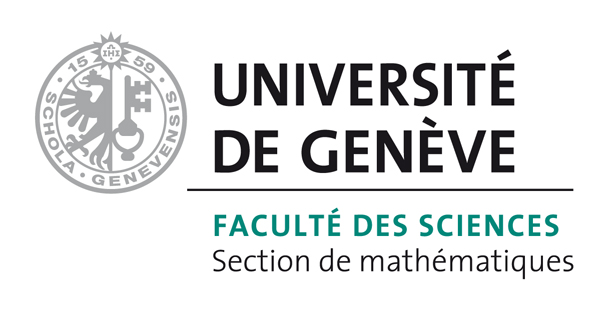# Bart Vandereycken## A survey and comparison of contemporary algorithms for computing the matrix geometric mean

by , ,

#### Abstract:

In this paper we present a survey of various algorithms for computing matrix geometric means and derive new second-order optimization algorithms to compute the Karcher mean. These new algorithms are constructed using the standard definition of the Riemannian Hessian. The survey includes the ALM list of desired properties for a geometric mean, the analytical expression for the mean of two matrices, algorithms based on the centroid computation in Euclidean (flat) space, and Riemannian optimization techniques to compute the Karcher mean (preceded by a short introduction into differential geometry). A change of metric is considered in the optimization techniques to reduce the complexity of the structures used in these algorithms. Numerical experiments are presented to compare the existing and the newly developed algorithms. We conclude that currently first-order algorithms are best suited for this optimization problem as the size and/or number of the matrices increase.

#### Reference:

B. Jeuris, R. Vandebril, B. Vandereycken, "A survey and comparison of contemporary algorithms for computing the matrix geometric mean", In ETNA, vol. 39, pp. 379-402, 2012.

#### Bibtex Entry:

@article{JeurisVV_2012,
Abstract = {In this paper we present a survey of various algorithms for computing matrix geometric means and derive new second-order optimization algorithms to compute the Karcher mean. These new algorithms are constructed using the standard definition of the Riemannian Hessian. The survey includes the ALM list of desired properties for a geometric mean, the analytical expression for the mean of two matrices, algorithms based on the centroid computation in Euclidean (flat) space, and Riemannian optimization techniques to compute the Karcher mean (preceded by a short introduction into differential geometry). A change of metric is considered in the optimization techniques to reduce the complexity of the structures used in these algorithms. Numerical experiments are presented to compare the existing and the newly developed algorithms. We conclude that currently first-order algorithms are best suited for this optimization problem as the size and/or number of the matrices increase.},
Author = {Jeuris, B. and Vandebril, R. and Vandereycken, B.},
Journal = {ETNA},
Pages = {379--402},
Pdf = {http://www.unige.ch/math/vandereycken/papers/published_JeurisVV_2012.pdf},
Title = {A survey and comparison of contemporary algorithms for computing the matrix geometric mean},
Url = {http://etna.mcs.kent.edu/volumes/2011-2020/vol39/abstract.php?vol=39&pages=379-402},
Volume = {39},
Year = {2012}}